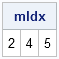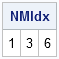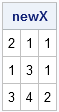Missing values are a fact of life. Many statistical analyses, such as regression, exclude observations that contain missing values prior to forming matrix equations that are used in the analysis. This post shows how to find rows of a data matrix that contain missing values and how to remove those rows.

Suppose that your data consist of three numerical variables and that missing values can occur for any variable. For example:

```proc iml; /** define 6 x 3 data matrix; rows 2, 4, and 5 contain missing values **/ x = {2 1 1, 4 . 1, 1 3 1, . 6 1, 1 1 ., 3 4 2};```

In SAS software, most procedures automatically handle missing values in the data. However, if you are using PROC IML to, for example, form the x`x matrix as part of a regression analysis, you need to manually locate and remove missing values in the data matrix before you can begin matrix multiplication.

The most common way of dealing with missing values is to remove an entire row (observation) if there is a missing value anywhere in that row. Therefore, the first step in an analysis is often to find the rows that have a missing value for any variable. For our example, we want to find rows 2, 4, and 5.

### Find Observations That Contain Missing Values

The straightforward approach is to loop over all variables. For each variable, use the LOC function to find the observations that are missing:

```/** Find observations that have a missing value for any variable **/ /** Version 1: Use a loop over columns **/ do j = 1 to ncol(x); /** loop over all columns **/ colIdx = loc(x[,j]=.); /** find indices of obs that are missing **/ mIdx = union(mIdx, colIdx);/** accumulate the indices **/ end; print mIdx;```That's not a bad approach, but you can actually avoid the loop if you remember that you can call Base SAS functions from the SAS/IML language. If you pass a matrix to the CMISS function, you get back a matrix of zeros and ones, where a 1 indicates that the corresponding entry of the x matrix is missing. You can then use the summation subscript operator (+) to count, for each row, how many missing values are in the row.

```/** Version 2: Use the CMISS function **/ c = cmiss(x); /** matrix of zeros and ones **/ count = c[,+]; /** add across rows **/ mIdx = loc(count>0); /** find rows with one or more missing values **/```

### Find Observations That Contain Only Nonmissing Values

The mIdx vector contains the indices of the rows that have a missing value for some variable. Sometimes you need the complement of this vector: the indices of the rows for which no variable is missing. You can use the SETDIF function to find the complement:

```NMIdx = setdif( 1:nrow(x), mIdx ); /** find nonmissing rows **/ print NMIdx;```(You can also compute NMIdx directly: NMIdx = loc(count=0);)

To create the new (nonmissing) data matrix, use the NMIdx vector to subset the rows of the original matrix:

```newX = x[NMIdx,]; /** keep rows for which all obs are nonmissing **/ print newX;```You can now use newX in any matrix computation.

ShareDistinguished Researcher in Computational Statistics

Rick Wicklin, PhD, is a distinguished researcher in computational statistics at SAS and is a principal developer of SAS/IML software. His areas of expertise include computational statistics, simulation, statistical graphics, and modern methods in statistical data analysis. Rick is author of the books Statistical Programming with SAS/IML Software and Simulating Data with SAS.

1.Now that's clever! This is much more efficient than the double loop that I was using. Nice tip.

2.The first solution is one that I would not have thought of. The UNION function is one that I was not aware of, but is certainly good to know.

For solution #2, I would note that there is no need to use the CMISS function (which was made available only with SAS V9.2). The NMISS function will work just fine in place of the CMISS function in this example. The CMISS function allows testing for missing values of either character or numeric. An IML matrix can be only all character or all numeric. In the present case, we are testing a numeric matrix. For a numeric matrix in IML, the NMISS function which finds numeric missing values will work across all SAS versions.

I would offer an alternative to use of the NMISS or CMISS function. For numeric missing, we can write:

c = (x = .); /** matrix of missing indicators **/
count = c[,+];
mIdx = loc(count>0);

This can be modified for a character matrix as:

c = (x = " "); /** matrix of missing indicators **/
count = c[,+];
mIdx = loc(count>0);

This approach constructs exactly the same indicator matrix C as is obtained using the NMISS or CMISS functions. Since we don't invoke a (data step) function, I would anticipate that this solution would be more efficient.

3.I like it!

4.Another helpful post! I just implemented this into a macro I was working on. Thanks